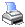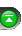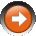﻿ Bollinger Bands
 NinjaScript > Language Reference > Indicator Methods > Bollinger BandsDescription

Developed by John Bollinger, Bollinger Bands are an indicator that allows users to compare volatility and relative price levels over a period time. The indicator consists of three bands designed to encompass the majority of a security's price action.

 1 A simple moving average in the middle
 2 An upper band (SMA plus 2 standard deviations)
 3 A lower band (SMA minus 2 standard deviations)

Standard deviation is a statistical unit of measure that provides a good assessment of a price plot's volatility. Using the standard deviation ensures that the bands will react quickly to price movements and reflect periods of high and low volatility. Sharp price increases (or decreases), and hence volatility, will lead to a widening of the bands.

... Courtesy of StockCharts

Syntax

 Bollinger(double numStdDev, int period) Bollinger(IDataSeries input, double numStdDev, int period)   Returns upper band value Bollinger(double numStdDev, int period).Upper[int barsAgo] Bollinger(IDataSeries input, double numStdDev, int period).Upper[int barsAgo]   Returns lower band value Bollinger(double numStdDev, int period).Lower[int barsAgo] Bollinger(IDataSeries input, double numStdDev, int period).Lower[int barsAgo]

Return Value

double; Accessing this method via an index value [int barsAgo] returns the indicator value of the referenced bar.

Parameters

 input Indicator source data (?) period Number of bars used in the calculation

Examples

 // Prints the current upper band value of a 20 period Bollinger using default price type double upperValue = Bollinger(2, 20).Upper; Print("The current Bollinger upper value is " + upperValue.ToString());   // Prints the current upper band value of a 20 period Bollinger using low price type double upperValue = Bollinger(Low, 2, 20).Upper; Print("The current Bollinger upper value is " + upperValue.ToString());

Source Code

You can view this indicator method source code by selecting the menu Tools > Edit NinjaScript > Indicator within the NinjaTrader Control Center window.